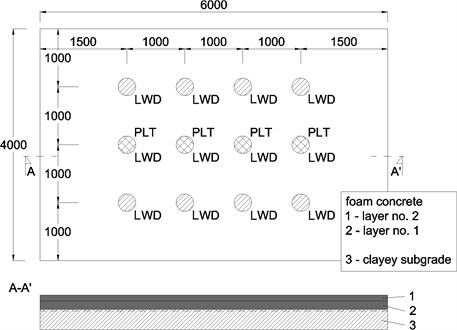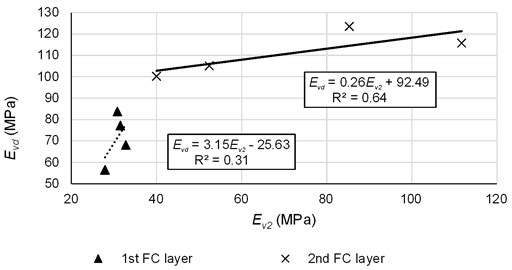Vibroengineering PROCEDIA

Published: 25 June 2019

# Dynamic testing of the sub-base layer made from foam concrete using light weight deflectometer

Jozef Vlcek2
Filip Gago3
Marian Drusa4
1, 2, 3, 4University of Zilina, Zilina, Slovakia
Corresponding Author:
Views 157

#### Abstract

Foam concrete (FC) is little used material in construction practice and it has very good potential for utilization in the civil engineering and building construction. Nowadays, it is primarily used as a leveling layer in the floor of the buildings, but its utilizability is much wider because of its particular properties. Its main characteristics are its material property and that it consists of void pores. This allows to achieve the low bulk density and saving of material inputs. As a building material, it has adequate mechanical characteristics and low thermal conductivity. A series of dynamic testing using light weight deflectometer (LWD) was carried out to estimate the reliable value of the dynamic deformation modulus that can represent the quality of the sub-base made from foam concrete beside the bulk density investigation. Static strain moduli from the second load cycle is the common required value for the sub-base design. Due to larger stiffness and thus larger deflection radius, conventional PLT apparatus is not suitable for foam concrete testing. On the other hand, when adequate dynamic modulus based on the conventional strain modulus is determined, LWD testing is quick method for the foam concrete testing. This paper presents the first attempts to estimate this relation.

## 1. Introduction

Foam concrete (FC) is a mixture of cement, water, additives and technical foam. FC is a building material with good mechanical and thermal properties, and fully mechanized preparation [1, 2]. It contains closed pores filled with air what allows to achieve low volume density and saving of material inputs. It can substitute the conventional sub-base layers of the industrial floors or pavements or it can be used as a part of the foundation of the buildings [3-6]. For the required bulk density, a specific formula can be developed. Typical bulk densities of the foam concrete start from 900 kg·m-3 [7, 8]. Further development of the formula allowed us to reduce the bulk density and conserve the mechanical properties such as compressive and tensile strength. The bulk densities then vary from 300 to 900 kg·m-3.

Utilization of the foam concrete as a pavement sub-base is one of the main topics of the research. Foam concrete was used several times as a part of the embankment body in case of soft subgrade due to its low bulk density in comparison to the conventional fill materials . In our proposal, foam concrete is used as a regular part of the pavement structure – sub-base layer with defined characteristics and boundary conditions as a substitution of the conventional bounded and unbounded materials.

## 2. Foam concrete testing

Requirements for the sub-base layer are same for both the conventional and foam concrete layer. In this paper a strain modulus and dynamic deformation modulus were investigated as a most common way to verify the quality of the sub-base. For granular materials, determination of the bulk density is the most accurate method to examine the level of compaction and homogeneity of the layer but this method is time consuming [10, 11]. Bulk density inspection is the mandatory part of the production process of the foam concrete to check the homogeneity of the mixture.

For the measurements a real scale physical model of the pavement sub-base and subgrade was prepared. Physical simulation is reliable method for investigation of the behavior of the structure. Together with numerical simulation, it creates a handful tool for the research and design purposes [12-14].

A series of tests at the experimental field was performed to evaluate the key properties of the sub-base made from foam concrete. In this case, static plate load test (PLT) and dynamic testing using light weight deflectometer (LWD) were adopted. LWD testing was selected because of quick execution and evaluation of the dynamic deformation modulus. PLT testing was performed to define the dependency of the static and dynamic moduli. This method requires beam with the gauges which can be affected by the large radius of the depression below the plate. LWD test is not affected by this phenomenon and thus is more suitable for the practical application.

Foam concrete FC 400 with the nominal dry bulk density of 400 kg·m-3 was selected for testing as a balanced formula of the mechanical and thermal properties.

### 2.1. Experimental field

Experimental field represents the sub-base of typical semi-rigid pavement structure on the subgrade. Observation can be then carried out at known boundary conditions to obtain relevant outputs. Configuration of the field is showed on Fig. 1. Subgrade was created with the antropogenous clay of intermediate plasticity with stiff consistency. Geotextile of unit weight of 200 g·m-2 was placed as regular component of the foam concrete layer. A total of 2 foam concrete layers were prepared, first with nominal thickness of 12 cm and second one with the thickness of 10 cm. Overall thickness of the foam concrete slab was 22 cm.

Fig. 1A schematic diagram of the experimental field. Circles represents the sites for LWD and PLT testing, dimensions in mmPLT and LWD testing was then carried out on the subgrade and on each of the foam concrete layers according to the scheme in Fig. 1. A total of 3 LWD test sites related to the central PLT testing point were proposed. PLT testing was made in accordance with the DIN standard . As a information about subgrade stiffness and homogeneity, strain modulus ${E}_{v2}$ was determined. It varied from 11.5 to 13.4 MPa.

### 2.2. Light weight deflectometer (LWD)

Light Weigh Deflectometer (LWD) apparatus inflicts the impact load on the tested layer. Falling weight of the weight of 10 kg leading by the steel rod is falling from the height of 0.755 m and hits the damping pad attached to the circular steel plate of the diameter $d=$ 0.3 m. The impact imposes a contact stress of 100 kPa (the force of 7.07 kN). The duration of the impact is 17.9 ms. This stress imposes the deflection of the surface of the tested layer. The dynamic deformation moduli ${E}_{vd}$ is calculated according to the Eq. (1):

1
${E}_{vd}=\frac{F}{d\bullet y}\left(1-{\mu }^{2}\right),$

where: ${E}_{vd}$ dynamic deformation moduli (MPa), $F$ impact force (= 7.07 kN), $d$ diameter of the steel plate (= 0.3 m), $y$ deflection under the steel plate (mm), $\mu$ Poisson’s ratio of tested compound (= 0.40 for the subgrade and 0.25 for the foam concrete FC 400).

Final read-out of the modulus ${E}_{vd}$ and deflection $y$ is done on the display of the electronic unit after 4th test impact. This value represents the response of the sub-base to the pass of the heavy truck wheel.

## 3. Results and discussion

The following figure shows relation between static strain modulus from second load cycle ${E}_{v2}$ and dynamic deformation modulus ${E}_{vd}$. Dynamic modulus was calculated as an average value from 3 test points (Fig. 1). Both of the modulus was rectified according to the actual foam concrete layer thickness at given test point.

Fig. 2Relation between static and dynamic deformation modulusFor the first 12 cm layer, results show large dispersion of the dynamic modulus. Despite the homogeneity of the static values, this dispersion is caused by the membrane like behavior of the foam concrete slab and the imperfections of the clayey subgrade. Porous nature of the foam concrete can be another factor.

Additional FC layer brings better relation, but static values of the modulus are more spread in this case because of small cracks that occurred during the test. These cracks also influenced the values of dynamic modulus which are lower.

## 4. Conclusions

Dynamic testing shows relative small reliability of achieved results. High porosity of the foam concrete with some damping potential complicate the estimation of the dynamic modulus as a replacement of the static PLT testing. Another testing is required to determine the reliable dynamic modulus ${E}_{vd}$ as a control parameter for the quality assessment. Porous composition of the foam concrete brings the problem to reliable estimate the dynamic properties of such a material. A large set of data should be collected and examined to determine the objectified value of the dynamic deformation modulus.

Next step will involve the numerical modelling to investigate the behavior of the foam concrete sub-grade under the PLT and LWD testing via parametric study at known static parameters of the foam concrete determined in the laboratory.

#### Acknowledgements

This article was prepared with the financial support of KEGA MŠVVaŠ SR Cultural and Educational Agency in the framework of grant No. 051ŽU-4/2018.

#### Cited by

Experimental and Numerical Verification of the Railway Track Substructure with Innovative Thermal Insulation Materials